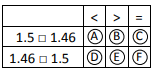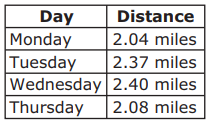# Standard #: MAFS.4.NF.3.7 (Archived Standard)

This document was generated on CPALMS - www.cpalms.org

Compare two decimals to hundredths by reasoning about their size. Recognize that comparisons are valid only when the two decimals refer to the same whole. Record the results of comparisons with the symbols >, =, or <, and justify the conclusions, e.g., by using a visual model.

### General Information

Subject Area: Mathematics
Domain-Subdomain: Number and Operations - Fractions
Cluster: Level 2: Basic Application of Skills & Concepts
Cluster: Understand decimal notation for fractions, and compare decimal fractions. (Major Cluster) -

Clusters should not be sorted from Major to Supporting and then taught in that order. To do so would strip the coherence of the mathematical ideas and miss the opportunity to enhance the major work of the grade with the supporting clusters.

Date of Last Rating: 02/14
Status: State Board Approved - Archived
Assessed: Yes

### Test Item Specifications

N/A

Assessment Limits :
Decimals may reference the same whole entity. Decimals are limited to tenths and hundredths. Decimals may be greater than 1. Items may not require a comparison of visual models in isolation.
Calculator :

No

Context :

Allowable

### Sample Test Items (4)

 Test Item # Question Difficulty Type Sample Item 1 Mr. Shelby bought a new plant. The plant grew 2.6 centimeters in the first week and 3.42 centimeters the second week.Select all the true comparisons of the plant growth for the two weeks. N/A MS: Multiselect Sample Item 2 Zach and Karla each have seeds they will plant in a class garden. Zach’s seeds weigh 1.5 grams. Karla’s seeds weigh 1.46 grams.Fill in the circles to select the correct symbol for each comparison.N/A MI: Matching Item Sample Item 3 Allison wrote down a decimal number that is greater than 0.58 but less than 0.62. What is one number Allison could have written down? N/A EE: Equation Editor Sample Item 4 The table shows the distances that Brianna ran on four days.Which comparison about the distances is true? N/A MC: Multiple Choice

#### Related Courses

 Course Number1111 Course Title222 5012060: Mathematics - Grade Four (Specifically in versions: 2014 - 2015, 2015 - 2022, 2022 and beyond (current)) 7712050: Access Mathematics Grade 4 (Specifically in versions: 2014 - 2015, 2015 - 2018, 2018 - 2022, 2022 and beyond (current)) 5020110: STEM Lab Grade 4 (Specifically in versions: 2016 - 2022, 2022 and beyond (current)) 5012065: Grade 4 Accelerated Mathematics (Specifically in versions: 2019 - 2022, 2022 and beyond (current)) 5012015: Foundational Skills in Mathematics 3-5 (Specifically in versions: 2019 - 2022, 2022 and beyond (current))

#### Formative Assessments

 Name Description Using Models to Compare Decimals Students are asked to compare decimals by drawing a visual model and record the comparison using the less than, greater than, or equal to symbol. Comparing Decimals in Context Students are asked to compare two pairs of decimals in the context of word problems and to record a comparison using an inequality symbol. Comparing Four Tenths Students are asked to consider two grids with different sized wholes and determine if both models show four-tenths. Compare Decimals Students are asked to compare four pairs of decimals using the less than, greater than, or equal to symbols.

#### Original Student Tutorial

 Name Description Return to Deciland: Locating Decimals on a Number Line Learn how to locate decimals on a number line and compare decimals to save the Decis from a wizard's spell in this interactive tutorial.

 Name Description Using Place Value Each part of this task highlights a slightly different aspect of place value as it relates to decimal notation. More than simply being comfortable with decimal notation, the point is for students to be able to move fluidly between and among the different ways that a single value can be represented and to understand the relative size of the numbers in each place.

#### Tutorial

 Name Description Comparing Two Decimals with a Visual Model In this Khan Academy tutorial video two decimals are compared using grid diagrams.

#### Virtual Manipulative

 Name Description Fraction Models An interactive tool to represent a fraction circle, rectangle, or set model with numerators and denominators ranging from 1 to 100. The decimal and percent equivalents of the created fraction are also displayed.

#### Original Student Tutorial

 Name Description Return to Deciland: Locating Decimals on a Number Line: Learn how to locate decimals on a number line and compare decimals to save the Decis from a wizard's spell in this interactive tutorial.

 Name Description Using Place Value: Each part of this task highlights a slightly different aspect of place value as it relates to decimal notation. More than simply being comfortable with decimal notation, the point is for students to be able to move fluidly between and among the different ways that a single value can be represented and to understand the relative size of the numbers in each place.

#### Tutorial

 Name Description Comparing Two Decimals with a Visual Model: In this Khan Academy tutorial video two decimals are compared using grid diagrams.